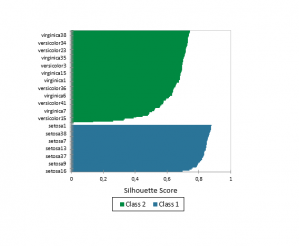# DBSCAN (Density-Based Spatial Clustering of Applications with Noise)

Use this method to perform anomaly detection and clustering on a set of observations described by quantitative and/or qualitative variables. Available in Excel using the XLSTAT software.## What is DBSCAN ?

DBSCAN stands for Density-based Spatial Clustering of Applications with Noise proposed by Ester, Kriegel, Sander and Xu in 1996. It is the most widely used unsupervised learning method among density-based clustering methods. There are several advantages to using this type of method: the ability to create an unknown number of classes, to create classes with non-convex shapes and the ability to handle anomalies.

In order to use the DBSCAN method, 2 parameters are required:

• ϵ >0 ;
• The number of minimum points, also called MinPts > 0

Several definitions allow us to understand how classes are made. First, we must define and count neighbors for each point. A neighbor is defined as any point p from the training dataset with a distance less than or equal to ϵ from a point q.

Note that by definition the point q is its own neighbor.

3 types of points can be defined with the DBSCAN algorithm:

• Core point: A point with as many or more neighbors as the number of minimum points.
• Border point: A point that has fewer neighbors than the number of minimum points but is a neighbor of a core point.
• Noise point: Neither a core point nor a border point.

A point p is directly density-reachable from q, if is a core point and p a neighbor of q. A point p is density-reachable from q if there is an ordered sequence of points directly density-reachable from the previous point. Two points p and q are density-connected if there is a point o such that both p and q are density-reachable from o.

Finally, Ester et al. defined a class as a subset of the dataset that fits two conditions:

• If p belongs to the class and is density-reachable from p then q belongs to C.
• All points in the class C are mutually density-connected.

### The DBSCAN algorithm

The DBSCAN algorithm visits all points of the training dataset and marks them visited as it goes.

If a point is a core point, then the first class is started (named class 1). The core point and its neighbors are assigned to class 1. Then, the algorithm visits its neighbors in order to find another core point and assigns it to class 1. This step allows the class to expand. The algorithm stops to expand class 1 when all density-reachable points have been visited.

The algorithm continues to visit the unvisited points and will start a new class if another core point is found. This class can also be expanded and so on...

Finally, all points which are not assigned to a class are noise points.

## Options for DBSCAN clustering in XLSTAT

### Prediction with DBSCAN

DBSCAN allows you to predict the class of new observations.

First, it must find the neighbors of each new observation in the training dataset. If a new observation is a neighbor of a core point (from the training dataset) then the new observation is assigned to the same class as the core point.

If the new observation has no core point in its neighbors, then it is considered as a noise point

Note that the visiting order can change the class assigned to the border points during learning and prediction.

### K-dimensional tree

Use the K-dimensional tree when the dataset contains only quantitative variables (Bentley, 1975). It makes it possible to not compute all the distances to find all the neighbors in a radius of size epsilon.

The k-dimensional tree is a binary tree constructed by sorting the points from one dimension and dividing the space into 2 from the median. Points with a value less than or equal to the median in this dimension are stored in the left child node while points with a value greater than the median are stored in the right child node. The construction of the tree stops when there is only one point left in a node.

### Distance metrics

There are different distance metrics to compute distances with any type of variable.

There are 5 metrics when only quantitative variables are selected:

• Euclidean distance
• Minkowski distance
• Manhattan distance
• Chebychev distance
• Canberra distance.

When only qualitative variables describe the observations, the Overlap distance is used.

With mixed data, the HEOM (Heterogeneous Euclidean Overlap Metric) is used.

## Results for DBSCAN clustering in XLSTAT

Descriptive statistics: The table of descriptive statistics shows the simple statistics for all the variables selected. The number of missing values, the number of non-missing values, the mean and the standard deviation (unbiased) are displayed for the quantitative variables. For qualitative variables, including the dependent variable, the categories with their respective frequencies and percentages are displayed.

Correlation matrix: This table is displayed to give you a view of the correlations between the various variables selected.

Number of objects by class: This table is displayed to give you a view of the size of each class and the number of noise points.

Results regarding distance matrices: One or two distance matrices are displayed if the prediction option is activated. The first matrix shows distances between each point of the training sample. The second matrix shows distances between the new observations and the observations of the training sample.

Results regarding objects: Classes assigned to each observation using the DBSCAN algorithm are displayed for the training and the prediction sample. If the class is 0 it means the observation is considered as a noise point. In addition, the silhouette score of each observation is displayed in the second column (if the option is activated).

A graph of the silhouette scores is displayed if the option is activated. Observations are grouped by classes in descending order with respect to the silhouette coefficient.

Results associated with objects sorted by class: this table is displayed to show the observations sorted by class.### analysieren sie ihre daten mit xlstat

kostenlose 14-tage-testversion

Enthalten ind

Verwandte Funktionen